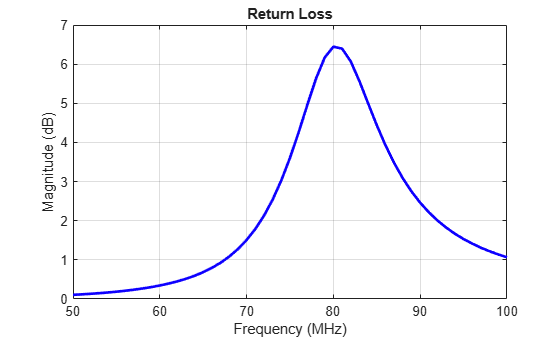# returnLoss

Return loss of antenna or scan return loss of array

## Syntax

``returnLoss(antenna,frequency)``
``returnLoss(antenna,frequency,z0)``
``rl = returnLoss(antenna,frequency,z0)``
``returnLoss(array,frequency,z0,elementnumber)``
``rl = returnLoss(array,frequency,z0,elementnumber)``

## Description

````returnLoss(antenna,frequency)` calculates and plots the return loss of an antenna, over a specified frequency and at a reference impedance of 50 ohm.```

example

````returnLoss(antenna,frequency,z0)` calculates and plots the return loss of an antenna, over a specified frequency and a given reference impedance, `z0`.```
````rl = returnLoss(antenna,frequency,z0)` returns the return loss of an antenna.```
````returnLoss(array,frequency,z0,elementnumber)` calculates and plots the scan return loss of a specified antenna element in an array.```
````rl = returnLoss(array,frequency,z0,elementnumber)` returns the scan return loss of a specified antenna element in an array. ```

## Examples

collapse all

This example shows how to calculate and plot the return loss of a circular loop antenna over a frequency range of 50MHz-100MHz.

```h = loopCircular; returnLoss (h, 50e6:1e6:100e6);```## Input Arguments

collapse all

Antenna to calculate return loss, specified as either:

Example: `dipole`

Example: `customAntennaMesh`

Example: `customAntenna`

Example: `pcbStack`

Array to calculate scan return loss, specified as either:

Example: `linearArray`

Example: `customArrayGeometry`

Example: `array(pcbStack,"linear")`

Frequency range used to calculate return loss, specified as a vector in Hz.

Example: 50e6:1e6:100e6

Data Types: `double`

Reference impedance, specified as a scalar in ohms.

Example: 40

Data Types: `double`

Antenna element number in array, specified as a scalar.

Example: `1`

Data Types: `double`

## Output Arguments

collapse all

Return loss of antenna or scan return loss of array, returned as a vector in dB. The return loss is calculated using the formula

`$RL=-20{\mathrm{log}}_{10}\text{ }\text{\hspace{0.17em}}|\frac{\left(Z-{Z}_{0}\right)}{\left(Z+{Z}_{0}\right)}|$`

where,

• Z = input impedance of antenna or scan impedance of array

• Z0 = reference impedance

## Version History

Introduced in R2015a